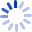# Introduction to Calculus and Analysis II/1

From the reviews "These books (Introduction to Calculus and Analysis Vol. I/II) are very well written. The mathematics are rigorous but the many examples that are given and the applications that are treated make the books extremely readable and the arguments easy to understand. These books are ideally suited for an undergraduate calculus course. Each chapter is followed by a number of interesting exercises. More difficult parts are marked with an asterisk. There are many illuminating figures...Of interest to students, ...• Book Details
• Seller